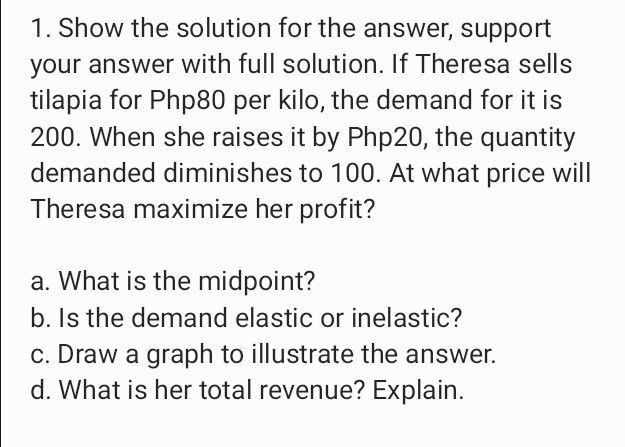### ¿Todavía tienes preguntas de matemáticas?

Pregunte a nuestros tutores expertos
Algebra
Pregunta1. Show the solution for the answer, support your answer with full solution. If Theresa sells tilapia for Php80 per kilo, the demand for it is $$200$$ . When she raises it by Php20, the quantity demanded diminishes to $$100$$ . At what price will Theresa maximize her profit? a. What is the midpoint? b. Is the demand elastic or inelastic? c. Draw a graph to illustrate the answer. d. What is her total revenue?

$$a \cdot ( \frac { 180 } { 2 } , \frac { 300 } { 2 } ) \Rightarrow ( 90,50 )$$
d. $$x y = - 5 x ^ { 2 } + 600 x = - 5 ( x ^ { 2 } - 120 x )$$$$= - 5 ( x - 60 ) ^ { 2 } + 18000$$
php $$=$$ 60,  maximize her profit